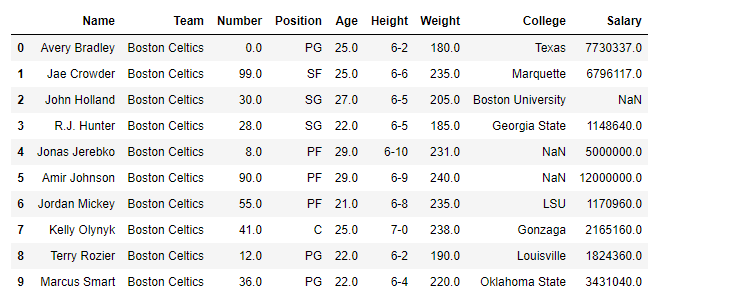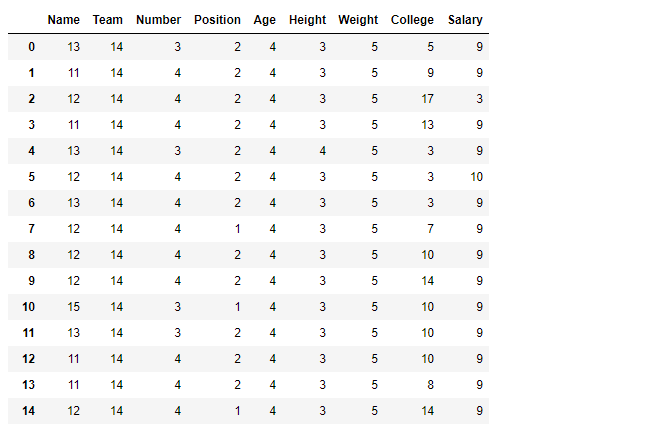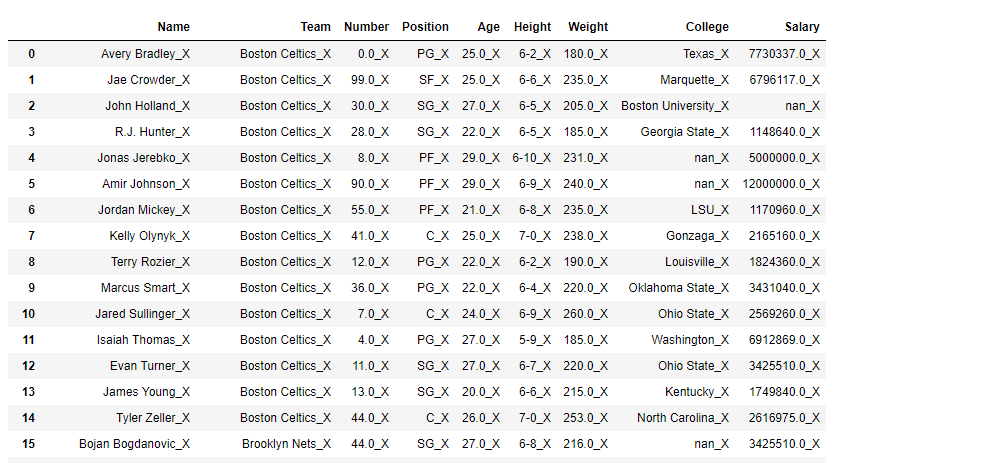GeeksforGeeks App
Open AppBrowser
Continue

# Python | Pandas dataframe.applymap()

Python is a great language for doing data analysis, primarily because of the fantastic ecosystem of data-centric python packages. Pandas is one of those packages and makes importing and analyzing data much easier.

`Dataframe.applymap()` method applies a function that accepts and returns a scalar to every element of a DataFrame.

```Syntax: DataFrame.applymap(func)

Parameters:
func: Python function, returns a single value from a single value.

Returns: Transformed DataFrame.
```

Example #1: Apply the `applymap()` function on the dataframe to find the no. of characters in all cells.

 `# importing pandas as pd``import` `pandas as pd`` ` `# Making data frame from the csv file``df ``=` `pd.read_csv(``"nba.csv"``)`` ` `# Printing the first 10 rows of ``# the data frame for visualization``df[:``10``]``# Using lambda function we first convert all ``# the cell to a string value and then find``# its length using len() function``df.applymap(``lambda` `x: ``len``(``str``(x)))`

Output:Notice how all nan value has been converted to string nan and their length is evaluated to be 3.

Example #2: Append `_X `in each cell using `applymap()` function.

In order to append `_X `in each cell, first convert each cell into a string.

 `# importing pandas as pd``import` `pandas as pd`` ` `# Making data frame from the csv file``df ``=` `pd.read_csv(``"nba.csv"``)`` ` `# Using applymap() to append '_X'``# in each cell of the dataframe``df.applymap(``lambda` `x: ``str``(x) ``+` `'_X'``)`

Output:My Personal Notes arrow_drop_up